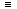1. It was Sunday on Jan 1, 2006. What was the day of the week Jan 1, 2010?

On 31st December, 2005 it was Saturday.

Number of odd days from the year 2006 to the year 2009 = (1 + 1 + 2 + 1) = 5 days.On 31st December 2009, it was Thursday.

Thus, on 1st Jan, 2010 it is Friday.

2.  What was the day of the week on 28th May, 2006?

28 May, 2006 = (2005 years + Period from 1.1.2006 to 28.5.2006)
Odd days in 1600 years = 0
Odd days in 400 years = 0

5 years = (4 ordinary years + 1 leap year) = (4 x 1 + 1 x 2)6 odd days
`Jan.  Feb.   March    April    May (31 +  28  +  31   +   30   +   28 ) = 148 days`148 days = (21 weeks + 1 day)1 odd day.

Total number of odd days = (0 + 0 + 6 + 1) = 70 odd day.
Given day is Sunday.

3.  What was the day of the week on 17th June, 1998?

17th June, 1998 = (1997 years + Period from 1.1.1998 to 17.6.1998)
Odd days in 1600 years = 0

Odd days in 300 years = (5 x 3)1
97 years has 24 leap years + 73 ordinary years.

Number of odd days in 97 years ( 24 x 2 + 73) = 121 = 2 odd days.
`Jan.  Feb.   March    April    May      June (31 +  28  +  31   +   30   +   31   +   17) = 168 days`168 days = 24 weeks = 0 odd day.

Total number of odd days = (0 + 1 + 2 + 0) = 3.
Given day is Wednesday.

4. What will be the day of the week 15th August, 2010?

15th August, 2010 = (2009 years + Period 1.1.2010 to 15.8.2010)

Odd days in 1600 years = 0
Odd days in 400 years = 0

9 years = (2 leap years + 7 ordinary years) = (2 x 2 + 7 x 1) = 11 odd days4 odd days.
`Jan.  Feb.   March    April    May  June  July  Aug. (31 +  28  +  31   +   30   +  31  + 30  + 31  + 15) = 227 days`227 days = (32 weeks + 3 days)3 odd days.

Total number of odd days = (0 + 0 + 4 + 3) = 70 odd days.
Given day is Sunday.

5. Today is Monday. After 61 days, it will be:

Each day of the week is repeated after 7 days.
So, after 63 days, it will be Monday.After 61 days, it will be Saturday.